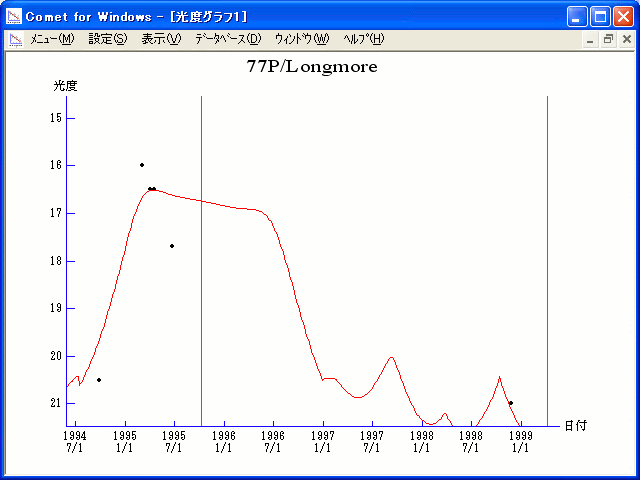# \$B%m%s%0%b%"WB@1(B

77P/Longmore (1995)###\$B50F;MWAG(B

```   The following improved orbital elements by Kenji Muraoka,
are from 107 observations 1975 to 1998, including 9 Planets,
Moon and 5 minor planets perturbations and non-gravitational
effect of style II.  The mean residual is +/- 0.75 arc seconds.

Epoch  =  1995 Oct. 10.0  TT       JDT = 2450000.5
T  =  1995 Oct.  9.31729       +/- 0.00183 (m.e.) TT
Peri. =  195.79660                +/- 0.00038
Node  =   15.65627                +/- 0.00008   (2000.0)
Incl. =   24.40989                +/- 0.00003
q  =    2.3989680              +/- 0.0000018 AU
e  =    0.3430595              +/- 0.0000004
a  =    3.6517281              +/- 0.0000009 AU
n  =    0.14123958             +/- 0.00000005
P  =    6.978                  +/- 0.0000026  years
A1  =   +0.089                  +/- 0.098
A2  =   -0.07111                +/- 0.00294
```

###\$B@1?^(B1994\$BG/(B10\$B7n(B 5\$BF|!A(B1995\$BG/(B11\$B7n(B29\$BF|(B1995\$BG/(B 8\$B7n(B21\$BF|!A(B1996\$BG/(B 9\$B7n(B24\$BF|(B

###\$B8wEYJQ2=(B

```        H = 14.0  G = 0.15               [    ,-450]  (              \$B!A(B1994\$BG/(B 7\$B7n(B16\$BF|(B)
m1 = 6.5 + 5 log\$B&\$(B + 20.0 log r  [-450, 450]  (1994\$BG/(B 7\$B7n(B16\$BF|!A(B1997\$BG/(B 1\$B7n(B 1\$BF|(B)
H = 14.0  G = 0.15               [ 450,    ]  (1997\$BG/(B 1\$B7n(B 1\$BF|!A(B              )
```##### \$B50F;MWAG\$OB<2,7r<#;a\$N7W;;\$K\$h\$k\$b\$N\$G\$9!#(B \$B@1?^\$O(B StellaNavigator Ver.2.0 for Windows (\$B%"%9%H%m%"!<%D(B \$BJTCx(B / \$B%"%9%-!<=PHG6I4)(B) \$B\$G:n@.\$7\$?\$b\$N\$G\$9!#(B \$B8wEY%0%i%U\$O(BComet for Windows\$B\$G:n@.\$7\$?\$b\$N\$G\$9!#(B